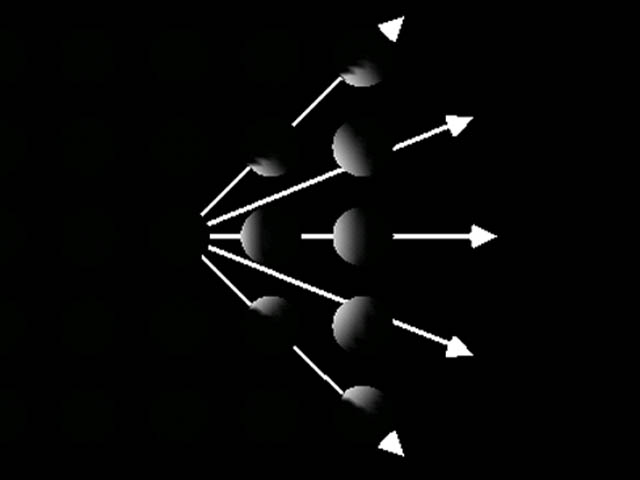# Spot Lights

###Method Default
`void setDirection( Vector3f dir )`
`0.0, 0.0, -1.0`
`void setSpreadAngle( float angle )`
`PI`
`void setConcentration( float concen )`
`0.0 `

• #### Concentrations vary from 0.0 (unfocused) to 128.0 (focused)

Direction - The axis of the cone of light. The default direction is (0.0, 0.0, -1.0). The spot light direction is significant only when the spread angle is not PI radians (which it is by default).
* Spread angle - The angle in radians between the direction axis and a ray along the edge of the cone. Note that the angle of the cone at the apex is then twice this value. The range of values is [0.0,PI/2] radians, with a special value of PI radians. Values lower than 0 are clamped to 0 and values over PI/2 are clamped to PI. The default spread angle is PI radians.
* Concentration - Specifies how quickly the light intensity attenuates as a function of the angle of radiation as measured from the direction of radiation. The light's intensity is highest at the center of the cone and is attenuated toward the edges of the cone by the cosine of the angle between the direction of the light and the direction from the light to the object being lit, raised to the power of the spot concentration exponent. The higher the concentration value, the more focused the light source. The range of values is [0.0,128.0]. The default concentration is 0.0, which provides uniform light distribution.

`TransformGroup group = new TransformGroup( );. . .SpotLight light = new SpotLight( );light.setEnable( true );light.setColor( new Color3f( 1.0f, 1.0f, 1.0f ) );light.setPosition( new Point3f( 0.0f, 1.0f, 0.0f ) );light.setAttenuation( new Point3f( 1.0f, 0.0f, 0.0f ) );light.setDirection( new Vector3f( 1.0f, 0.0f, 0.0f ) );light.setSpreadAngle( 0.785f );  // 45 degreeslight.setConcentration( 0.0f );  // Unfocused. . .light.setInfluencingBounds( bounds );group.addChild( light );`

 Web www.vrarchitect.net